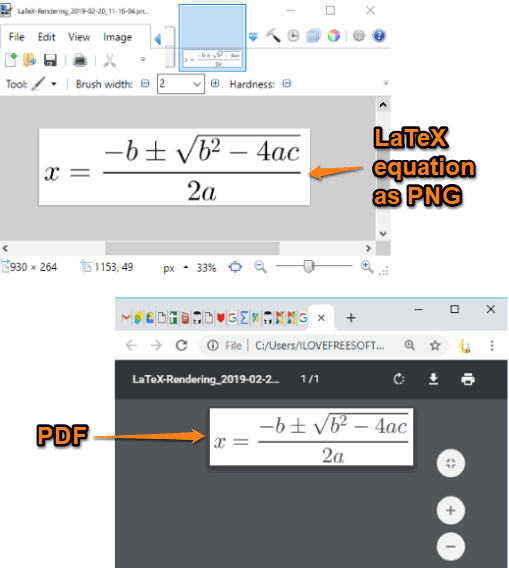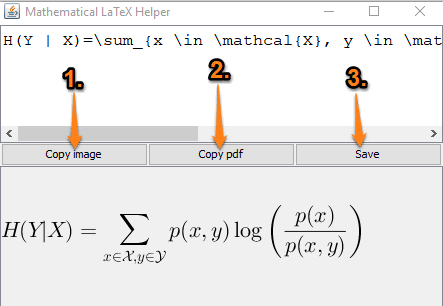Editor Ratings:
User Ratings:
[Total: 0 Average: 0]

In this tutorial, you will learn how to convert LaTeX to PNG image and PDF file. You will be able to add LaTeX equation as well as edit it and that equation is rendered automatically to generate the output image. Both the input equation and output image are visible at a time to get a clear idea about the output. All the changes added by you in the LaTeX equation are visible in real-time for the output image.

To convert LaTeX to PNG image and/or PDF, I am using very simple and free software. It is named “Mathematical LaTeX Helper“. It has a straightforward interface where you can put the LateX expression and get its output as PNG and PDF.In the screenshot above, you can see a PNG image for a LaTeX equation and PDF file for the same.

## How to Convert LaTeX to PNG Image and PDF Document?

Step 1: This software is available in a portable version as well as the installer version. You can use the link that I have added at the end of this article and then download any version.

Step 2: Open the software interface and then you can see a sample LaTeX equation.Step 3: You can continue with the existing equation or add a new equation. The output image is generated immediately on the bottom part of its interface. Along with that, whenever you edit the equation, the change is reflected in real-time. Now, to save the output image and PDF, first, click on the Copy image and Copy pdf buttons. After that, use the Save button.It will immediately create PNG and PDF files and store them on the desktop. There will be no watermark in the output files which makes it more useful.

### The Conclusion:

This is a simpler option to convert LaTeX to PNG image and PDF file. The good thing is you can edit the equation as many times as you want and changes are visible in real-time. The entire process is pretty simple.